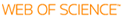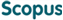### BROWSE

#### Related ResearcherKwon, Min-Suk
Ubiquitous Photonics Laboratory (UP Lab)
Research Interests
• Photonics, plasmonics, waveguides, planar lightwave circuits

A Numerically Stable Analysis Method for Complex Multilayer Waveguides Based on Modified Transfer-Matrix Equations

Cited 1 times inCited 1 times inTitle
A Numerically Stable Analysis Method for Complex Multilayer Waveguides Based on Modified Transfer-Matrix Equations
Author
Keywords
Characteristic equations; Iteration method; Multilayer waveguides; Transfer-matrix equations (TMEs)
Issue Date
2009-10
Publisher
IEEE-INST ELECTRICAL ELECTRONICS ENGINEERS INC
Citation
JOURNAL OF LIGHTWAVE TECHNOLOGY, v.27, no.20, pp.4407 - 4414
Abstract
This paper discusses a method of analyzing complex one-dimensional multilayer waveguides in a numerically stable way. When a multilayer waveguide contains a quite thick layer or a layer whose refractive index has a very large real or imaginary part, conventional analysis methods tend to fail to find out its modes. In order to solve such a problem, the discussed analysis method is based on modified transfer matrix equations. The method consists of three steps. At the first step, two types of modified transfer matrix equations are used to derive two types of characteristic equations. At the next step, the iteration method comprised of initial root-finding and root-tracking is used to find the roots of the characteristic equations. Since the two characteristic equations have different features, the initial root-finding is carried out with one of them, and the root-tracking with the other. Finally, the field profiles of waveguide modes are calculated by using the found roots and employing a stable routine based on the modified transfer matrix equations. A few presented examples show that the discussed method works well. In addition to the method, it is shown that one of the characteristic equations can be expressed as an explicit form rather than a conventional recursive matrix relation because of the simple form of one of the transfer matrix equations.
URI
DOI
10.1109/JLT.2009.2024089
ISSN
0733-8724
Appears in Collections:
EE_Journal Papers
Files in This Item: